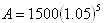Formula
Example 3

Peter earned \$1500 last summer. If he deposited the money in a bank account that earns 5% interest compounded yearly, how much money will he have after five years?

Step 1. Identify the variables.

A is the ending amount, this is what we are trying to determine.

P is the beginning amount, in this case 1500.

r is the interest rate written in the form of a decimal, in this case 0.05.

t is the time in years, in this case 5 years.

n is the number of times compounded per year, in this case it is being compounded yearly and therefore n = 1.

*Note: Since n = 1, it will not effect the equation and can be eliminated from the formula. In this case the general form of an exponential function ( y = abx) is just as effective at solving the problem.

Step 2. Substitute the variables into the formula.Step 3. Calculate the formula, you may need a calculator.

y = 1500 · (1.05)5 = \$1914.42

.